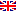Scheda programma d'esame
TEORIA ERGODICA
GIANLUIGI DEL MAGNO
CdSMATEMATICA
Codice224AA
CFU6
PeriodoPrimo semestre
LinguaItaliano

ModuliSettore/iTipoOreDocente/i
TEORIA ERGODICAMAT/05LEZIONI42
 GIANLUIGI DEL MAGNO unimap TANJA ISABELLE SCHINDLER unimapEsporta in pdf
Programma non disponibile nella lingua selezionata
Learning outcomes
Knowledge

The course will provide an introduction to the subject of ergodic theory. The student will learn the basic concepts and methods of ergodic theory, which help analyze dynamical systems in mathematics and other scientific disciplines.

Assessment criteria of knowledge
1. Homework
2. Final written essay and oral presentation on a topic in ergodic theory chosen by the student and approved by the lecturers
Skills

The student

• will have a detailed knowledge of a number of fundamental examples in ergodic theory
• will understand the proofs of the fundamental theorems
Assessment criteria of skills

Homework

Discussion of concepts and homework problems in class and during office hours

Behaviors

The student

• will be able to apply basic concepts and methods of ergodic theory for studying dynamical systems in mathematics and other scientific disciplines
• will have a solid background in order to understand current research problems in ergodic theory and possibly to undertake research in the area
Assessment criteria of behaviors

The student will have to show a full comprehension of the subjects presented in class and the ability to apply them to other situations.

Prerequisites

Real analysis, basic notions of measure theory and functional analysis

At least one half of the course will be lectured in english

Syllabus

Ergodic theory is the mathematical discipline dealing with dynamical systems endowed with invariant measures. A (discrete) dynamical system is a transformation f : M → M in some measurable space M. We think of M as the space of all possible states of a given system, and of f as the evolution law of the system. If the element x in M is the initial state of the system, then f(x) is the state of the system after of unit of time.  We always assume that f is measurable and leaves invariant a measure defined on M.

The topics covered in the course are the following:

1. Recurrence: invariant measures, Poincaré recurrence theorem, examples
2. Existence of invariant measures: weak* topology, Krylov-Bogoliubov theorem
3. Ergodic Theorems: von Neumann ergodic theorem, Birkhoff ergodic theorem, subadditive ergodic theorem
4. Ergodicity: ergodic systems, examples, properties of ergodic measures, ergodic decomposition
5. Unique ergodicity: unique ergodicity, minimality, Haar measures
6. Mixing: mixing systems, Markov shifts
7. Equivalent systems: ergodic equivalence, spectral equivalence
8. Entropy: metric entropy, Kolmogorov-Sinai theorem, local entropy, examples
9. Expanding maps: existence of absolutely continuous invariant measures
Bibliography
1. M. Viana and K. Oliveira, Foundations of Ergodic Theory, Cambridge University Press, 2016
2. P. Walters, An introduction to ergodic theory, Springer, 1982

Assessment methods
1. Homework
2. Final written essay on a topic chosen by student and approved by lecturers
3. Oral presentation of the final essay on Google Meet

The student may choose to write the homework, the final essay and deliver the oral presentation in italian or english.

Ultimo aggiornamento 11/09/2020 13:28